Courses

# Test: Geometry & Mensuration (2019-2010)

## 10 Questions MCQ Test UPSC Topic Wise Previous Year Questions | Test: Geometry & Mensuration (2019-2010)

Description
This mock test of Test: Geometry & Mensuration (2019-2010) for UPSC helps you for every UPSC entrance exam. This contains 10 Multiple Choice Questions for UPSC Test: Geometry & Mensuration (2019-2010) (mcq) to study with solutions a complete question bank. The solved questions answers in this Test: Geometry & Mensuration (2019-2010) quiz give you a good mix of easy questions and tough questions. UPSC students definitely take this Test: Geometry & Mensuration (2019-2010) exercise for a better result in the exam. You can find other Test: Geometry & Mensuration (2019-2010) extra questions, long questions & short questions for UPSC on EduRev as well by searching above.
QUESTION: 1

### A solid cube is painted yellow, blue and black such that opposite faces are of same colour. The cube is then cut into 36 cubes of two different sizes such that 32 cubes are small and the other four cubes are big. None of the faces of the bigger cubes is painted blue. How many cubes have only one face painted? 

Solution:

Number of cubes have only one face painted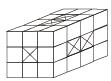= 2 × 4 + 2 × 1
= 8 + 2 = 10

QUESTION: 2

### Consider the following three-dimensional figure: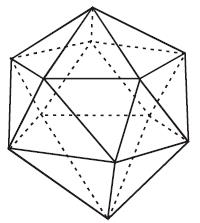How many triangles does the above figure have? 

Solution:

For easily count the number of triangles in the given three-dimensional figure, devide the given figure into three parts-top, bottom and middle.
In each of the top and bottom part, there are 5 triangles which are based on a pentagon. In middle part, there are 5 quadrilaterals inclosed between two pentagons.
Further each quadrilateral is divided into two triangles.
Hence in the middle part, there are 10 triangles.
∴ Total number of triangles in the given 3D-figure.
= (No. of triangles in top part) + (No. of triangle in bottom part) + (No. of triangle in middle part)
= 5+ 5 + 10 = 20

QUESTION: 3

### Twelve equal squares are placed to fit in a rectangle of diagonal 5 cm. There are three rows containing four squares each. No gaps are left between adjacent squares. What is the area of each square? 

Solution:

Diagonal of rectangle = 5 cm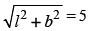then velue of l and b must be 4 and 3
Area of ractangle = 4 × 3 = 12 cm2
Area of square be a2
12 × a2 = 12
∴ a2 = 1 sq. cm.

QUESTION: 4

A piece of tin is in the form of a rectangle having length 12 cm and width 8 cm. This is used to construct a closed cube. The side of the cube is:



Solution:

Area of rectangle = 12 × 8 cm2 = 96 cm2
Rectangle is used to construct a closed cube.
Surface area of cube formed = Area of given rectangle.
6 (side)2 = 96 cm2
⇒ side =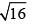⇒ side = 4 cm.

QUESTION: 5

A cylindrical overhead tank of radius 2 m and height 7 m is to be filled from an underground tank of size 5.5 m × 4 m × 6 m. How much portion of the underground tank is still filled with water after filling the overhead tank completely?



Solution:

Volume of cylindrical over head tank = πr2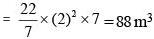Volume of underground tank = (5.5 × 4 × 6)m3 = 132m3
Portion of underground tank still filled with water after filling the overhead tank completely.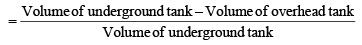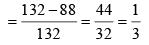QUESTION: 6

In a plane, line X is perpendicular to line Y and parallel to line Z; line U is perpendicular to both lines V and W; line X is perpendicular to line V.
Which one of the following statements is correct?



Solution: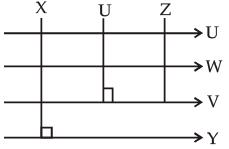From above diagram, it is clear that Y, V and W are parallel.

QUESTION: 7

A gardener increased the area of his rectangular garden by increasing its length by 40% and decreasing its width by 20%. The area of the new garden



Solution:

Let initial dimensions be, l & b
∴ Final length is 1.4 l
Final breadth is 0.8 b
∴ Final area is = 1.4 l × 0.8 b
= 1.12 lb
∴ Area is increased by 12%.
Shortcut Method : + 40 – 20 +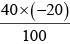= 20 – 8 = 12%
Therefore, the area of the new garden increased by 12%.

QUESTION: 8

Two glasses of equal volume are respectively half and three-fourths filled with milk. They are then filled to the brim by adding water. Their contents are then poured into another vessel. What will be the ratio of milk to water in this vessel?



Solution:

Milk in 1st Glass = (1/2)V
Milk in 2nd Glass = (3/4)V
Now the 1st glass = (1/2)V
Now the 2nd glass = (1/4)V
When both glasses are mixed then the ratio of milk to water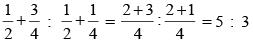QUESTION: 9

A village having a population of 4000 requires 150 litres of water per head per day. It has a tank measuring 20 m × 15 m × 6 m. The water of this tank will last for



Solution:

Volume of tank = 20 x 15 x 6 m3
= 20 x 15 x 6 x 1000 litre
∴ The water of the tank will last for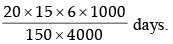i.e. The water of the tank will last for 3 days.

QUESTION: 10

The diameters of two circular coins are in the ratio of 1: 3. The smaller coin is, made to roll around the bigger coin till it returns to the position from where the, process of rolling started. How many times the smaller coin rolled around the bigger coin?



Solution:

Perimeter of bigger coin : Perimeter of smaller coin
= 2π(3) : 2π(1) = 3 : 1
Hence, smaller coin will roll 3 times around the bigger coin.